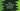# Java program to find three numbers in an array with total sum zero### Java Program to Find three numbers in an array with total sum zero :

In this tutorial, we will learn how to solve a common interview question known as 3SUM problem. The problem is one array is given and you have to find three numbers the sum of which is 0. For example, for the array (1,2,3,-4), the sum of 1,3 and -4 is 0. So it will print these three numbers.

### How to solve this problem :

We will learn three different methods to solve this problem. Let’s take a look at these methods one by one :

### Method 1 : Using Brute force method :

We will use three for loops to solve this problem.

• Outermost loop will run from i = 0 to i = length of the array.
• Inner loop will run from j = i+1 to j = length of the array.
• Third loop, which is the innermost loop will run from k = j+1 to k = length of the array.
• Inside of all these loops, we will check if the sum of all values for index i,j and k is zero or not. If yes, print out the values of the array.

Let’s take aa look at the program :

``````public class Main {

public static void main(String[] args) {
int givenArray[] = {1, 2, 4, -3, -1, 5, 6};

for (int i = 0; i < givenArray.length; i++) {
for (int j = i + 1; j < givenArray.length; j++) {
for (int k = j + 1; k < givenArray.length; k++) {
if (givenArray[i] + givenArray[j] + givenArray[k] == 0) {
System.out.println("Numbers found : " + givenArray[i] + "," + givenArray[j] + " and " + givenArray[k]);
}
}
}
}
}

}``````

It will print out the following output :

``````Numbers found : 1,2 and -3
Numbers found : 4,-3 and -1``````

### Complexity :

The time complexity is O(n^3).

### Method 2 : Using Hashing :

This method is better than the above one. We will use hashing table to solve this problem. Let’s try to understand how it works for an array :

• Use two inner for loops.
• The outer loop will run from i = 0 to the length of the array.
• The inner loop will run from j = i+1 to the length of the array.
• Create one HashSet. It will hold all the scanned numbers of the inner loop.
• Check if the ,-(value of i + value of j) exists in the hashset or not. If yes, means the sum of these three values is 0. Print out the results.
• Else, insert the current scanned value of the inner loop in the hashset.

Let’s take a look at the implementation of this problem :

``````import java.util.HashSet;

public class Main {

public static void main(String[] args) {
int givenArray[] = {1, 2, 4, -3, -1, 5, 6};
HashSet hashSet = new HashSet<>();

for (int i = 0; i < givenArray.length; i++) {
hashSet.clear();

for (int j = i + 1; j < givenArray.length; j++) {
int sum = -(givenArray[i] + givenArray[j]);
if(hashSet.contains(sum)){
System.out.println("Numbers found : " + givenArray[i] + "," + givenArray[j] + " and " + sum);
}

}
}
}

}``````

It will print :

``````Numbers found : 1,-3 and 2
Numbers found : 4,-1 and -3``````

### Complexity :

The complexity is better than the Method 1, O(n^2). But the problem is that we have to use extra space (HashSet) to solve this problem. Let’s try to solve this without using extra space :

### Method 3 : Using sorting :

We can also solve this problem by sorting the elements first. Steps :

• Sort the array.
• Start one for loop to run from i = 0 to i = length of the array.
• For each element of i, check other elements of the array,
• we will keep checking sum of two items of the remaining items. If the sum of these two and current element pointed by i is zero, print out the result.
• For this, we will use two pointers , one will point to the start and other will end of the array. If the current sum is less than zero, increment the value of start index. Else, increment the value of end index.

Let’s take a look at the program :

``````import java.util.*;

public class Main {

public static void main(String[] args) {
int givenArray[] = {1, 2, 4, -3, -1, 5, 6};
int firstElement;
int startIndex;
int endIndex;
int currentSum;

Arrays.sort(givenArray);

for (int i = 0; i < givenArray.length; i++) {
firstElement = givenArray[i];

startIndex = i + 1;
endIndex = givenArray.length - 1;

while (startIndex < endIndex) {
currentSum = givenArray[startIndex] + givenArray[endIndex];
if (currentSum + firstElement == 0) {
System.out.println("Found three elements " + firstElement + "," + givenArray[startIndex] + " and " + givenArray[endIndex]);
startIndex++;
endIndex--;
} else if (currentSum + firstElement < 0) {
startIndex++;
} else {
endIndex--;
}
}
}
}

}``````

It will print :

``````Found three elements -3,-1 and 4
Found three elements -3,1 and 2``````

### Complexity :

It has complexity same as Method 2 O(n^2), but this one is better because we are not using any extra space.